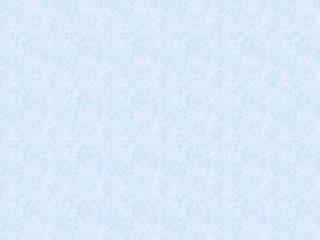DownloadDownload PresentationArea

# Area

Download Presentation## Area

- - - - - - - - - - - - - - - - - - - - - - - - - - - E N D - - - - - - - - - - - - - - - - - - - - - - - - - - -
##### Presentation Transcript

1. Area of Circle Area of Sector Area of Segment Segment Probability Area Probability 10 10 10 10 10 20 20 20 20 20 30 30 30 30 30 40 40 40 40 50 50 50 Area

2. Find the area of the circle. Leave your answer in terms of

3. Find the area of the circle. Leave your answer in terms of

4. Find the area of the circle. Use π = 3.14.

5. Find the area of the circle. Use π = 3.14.

6. The figure represents the overhead view of a deck surrounding a hot tub. What is the area of the deck? Round to the nearest tenth.

7. measure of arc 360° Find the area of the figure to the nearest tenth.

8. measure of arc 360° Find the area of a sector with a central angle of 180° and a diameter of 5.6 cm. Round to the nearest tenth.

9. measure of arc 360° Find the area of sector ZOM. Leave your answer in terms of π.

10. C 100° A Find the area of sector ACB. Leave your answer in terms of π. B 6 m

11. 50

12. Find the area of the shaded portion of the figure. Dimensions are in feet. Round your answer to the nearest tenth.

14. Find the area of the shaded segment. Round your answer to the nearest tenth. Find the area of sector AOB. Find the area of the segment. Find the area of ∆ AOB. The area of the segment is about 28.5 in.2

15. measure of arc 360° Find the exact area of the shaded region. 1.) Find the area of the sector. 2.) Find the area of the triangle. LL=√3•SL LL=12√3 3.) Subtract 30° 60° 24 597.2 - 144√3 597.2 - 249.4 348 hyp.=2•SL 24=2•SL 12=SL

16. A circle has a radius of 24 ft. Find the are of the smaller segment of the circle determined by a 120° arc. Round your answer to the nearest tenth. Find the area of the sector. 24 60° 120° 24 hyp.= 2•SL 24=2•SL 12=SL LL=√3•SL LL=√3•12 LL=12√3 Find the area of ∆ AOB. Find the area of the segment. The area of the segment is about 353.5 ft.2

17. Find the probability that a point chosen at random from is on the segment

18. Lenny’s favorite radio station has this schedule: news 13 min, commercials 2 min, music 45 min. If Lenny chooses a time of day at random to turn on the radio to his favorite station, what is the probability that the news will be on?

19. What is the probability that a point chosen at random on the grid will lie in the unshaded region?

20. A fly lands at a random point on the ruler's edge. Find the probability that the point is between 3 and 7. P(landing between 3 and 7) = = = 40 7-3=4

21. Anna's bus runs every 25 minutes. If she arrives at her bus stop at a random time, what is the probability that she will have to wait at least 10 minutes for the bus? Assume that a stop takes very little time, and let    represent the 25 minutes between buses. If Anna arrives at any time between A and C, she has to wait at least 10 minutes until B. P(waiting at least 10 min) =                      =      , or     The probability that Elena will have to wait at least 10 minutes for the bus is     or 60%.

22. If a dart hits the target at random, what it the probability that it will land in the shaded region?

23. The radius of the bull’s-eye of the dartboard is 8 inches. The radius of each concentric circle is 8 inches more than the radius of the circle inside it. If a dart lands at random on the dartboard, what is the probability that the dart will hit in area C?

24. Assume that a dart you throw will land on the 1-ft square dartboard and is equally likely to land at any point on the board. Find the probability of hitting each of the blue, yellow, and red regions. The radii of the concentric circles are 1, 2, and 3 inches, respectively. 30 The probabilities of hitting the blue, yellow, and red regions are about 2.2%, 6.5%, and 10.9%, respectively.

25. 40

26. 50